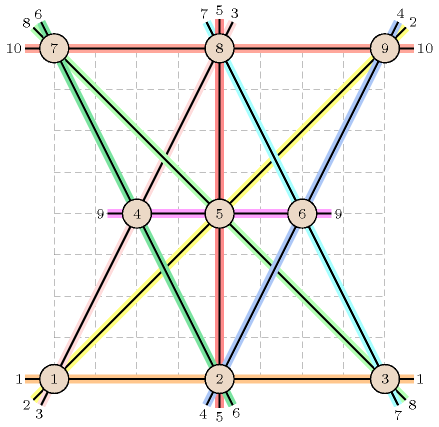## 5.304. orchard

Origin
Constraint

$\mathrm{𝚘𝚛𝚌𝚑𝚊𝚛𝚍}\left(\mathrm{𝙽𝚁𝙾𝚆},\mathrm{𝚃𝚁𝙴𝙴𝚂}\right)$

Arguments
 $\mathrm{𝙽𝚁𝙾𝚆}$ $\mathrm{𝚍𝚟𝚊𝚛}$ $\mathrm{𝚃𝚁𝙴𝙴𝚂}$ $\mathrm{𝚌𝚘𝚕𝚕𝚎𝚌𝚝𝚒𝚘𝚗}\left(\mathrm{𝚒𝚗𝚍𝚎𝚡}-\mathrm{𝚒𝚗𝚝},𝚡-\mathrm{𝚍𝚟𝚊𝚛},𝚢-\mathrm{𝚍𝚟𝚊𝚛}\right)$
Restrictions
 $\mathrm{𝙽𝚁𝙾𝚆}\ge 0$ $\mathrm{𝚃𝚁𝙴𝙴𝚂}.\mathrm{𝚒𝚗𝚍𝚎𝚡}\ge 1$ $\mathrm{𝚃𝚁𝙴𝙴𝚂}.\mathrm{𝚒𝚗𝚍𝚎𝚡}\le |\mathrm{𝚃𝚁𝙴𝙴𝚂}|$ $\mathrm{𝚛𝚎𝚚𝚞𝚒𝚛𝚎𝚍}$$\left(\mathrm{𝚃𝚁𝙴𝙴𝚂},\left[\mathrm{𝚒𝚗𝚍𝚎𝚡},𝚡,𝚢\right]\right)$ $\mathrm{𝚍𝚒𝚜𝚝𝚒𝚗𝚌𝚝}$$\left(\mathrm{𝚃𝚁𝙴𝙴𝚂},\mathrm{𝚒𝚗𝚍𝚎𝚡}\right)$ $\mathrm{𝚃𝚁𝙴𝙴𝚂}.𝚡\ge 0$ $\mathrm{𝚃𝚁𝙴𝙴𝚂}.𝚢\ge 0$
Purpose

Orchard problem [Jackson1821]:

Your aid I want, Nine trees to plant, In rows just half a score, And let there be, In each row, three—Solve this: I ask no more!

Example
$\left(\begin{array}{c}10,〈\begin{array}{ccc}\mathrm{𝚒𝚗𝚍𝚎𝚡}-1\hfill & 𝚡-0\hfill & 𝚢-0,\hfill \\ \mathrm{𝚒𝚗𝚍𝚎𝚡}-2\hfill & 𝚡-4\hfill & 𝚢-0,\hfill \\ \mathrm{𝚒𝚗𝚍𝚎𝚡}-3\hfill & 𝚡-8\hfill & 𝚢-0,\hfill \\ \mathrm{𝚒𝚗𝚍𝚎𝚡}-4\hfill & 𝚡-2\hfill & 𝚢-4,\hfill \\ \mathrm{𝚒𝚗𝚍𝚎𝚡}-5\hfill & 𝚡-4\hfill & 𝚢-4,\hfill \\ \mathrm{𝚒𝚗𝚍𝚎𝚡}-6\hfill & 𝚡-6\hfill & 𝚢-4,\hfill \\ \mathrm{𝚒𝚗𝚍𝚎𝚡}-7\hfill & 𝚡-0\hfill & 𝚢-8,\hfill \\ \mathrm{𝚒𝚗𝚍𝚎𝚡}-8\hfill & 𝚡-4\hfill & 𝚢-8,\hfill \\ \mathrm{𝚒𝚗𝚍𝚎𝚡}-9\hfill & 𝚡-8\hfill & 𝚢-8\hfill \end{array}〉\hfill \end{array}\right)$

The 10 alignments of 3 trees correspond to the following triples of trees: $\left(1,2,3\right)$, $\left(1,4,8\right)$, $\left(1,5,9\right)$, $\left(2,4,7\right)$, $\left(2,5,8\right)$, $\left(2,6,9\right)$, $\left(3,5,7\right)$, $\left(3,6,8\right)$, $\left(4,5,6\right)$, $\left(7,8,9\right)$. Figure 5.304.1 shows the 9 trees and the 10 alignments corresponding to the example.

##### Figure 5.304.1. Nine trees with 10 alignments of 3 treesTypical
 $\mathrm{𝙽𝚁𝙾𝚆}>0$ $|\mathrm{𝚃𝚁𝙴𝙴𝚂}|>3$
Symmetries
• Items of $\mathrm{𝚃𝚁𝙴𝙴𝚂}$ are permutable.

• Attributes of $\mathrm{𝚃𝚁𝙴𝙴𝚂}$ are permutable w.r.t. permutation $\left(\mathrm{𝚒𝚗𝚍𝚎𝚡}\right)$ $\left(𝚡,𝚢\right)$ (permutation applied to all items).

• One and the same constant can be added to the $𝚡$ attribute of all items of $\mathrm{𝚃𝚁𝙴𝙴𝚂}$.

• One and the same constant can be added to the $𝚢$ attribute of all items of $\mathrm{𝚃𝚁𝙴𝙴𝚂}$.

Arg. properties

Functional dependency: $\mathrm{𝙽𝚁𝙾𝚆}$ determined by $\mathrm{𝚃𝚁𝙴𝙴𝚂}$.

Keywords
Arc input(s)

$\mathrm{𝚃𝚁𝙴𝙴𝚂}$

Arc generator
$\mathrm{𝐶𝐿𝐼𝑄𝑈𝐸}$$\left(<\right)↦\mathrm{𝚌𝚘𝚕𝚕𝚎𝚌𝚝𝚒𝚘𝚗}\left(\mathrm{𝚝𝚛𝚎𝚎𝚜}\mathtt{1},\mathrm{𝚝𝚛𝚎𝚎𝚜}\mathtt{2},\mathrm{𝚝𝚛𝚎𝚎𝚜}\mathtt{3}\right)$

Arc arity
Arc constraint(s)
$\sum \left(\begin{array}{c}\mathrm{𝚝𝚛𝚎𝚎𝚜}\mathtt{1}.𝚡*\mathrm{𝚝𝚛𝚎𝚎𝚜}\mathtt{2}.𝚢-\mathrm{𝚝𝚛𝚎𝚎𝚜}\mathtt{1}.𝚡*\mathrm{𝚝𝚛𝚎𝚎𝚜}\mathtt{3}.𝚢,\hfill \\ \mathrm{𝚝𝚛𝚎𝚎𝚜}\mathtt{1}.𝚢*\mathrm{𝚝𝚛𝚎𝚎𝚜}\mathtt{3}.𝚡-\mathrm{𝚝𝚛𝚎𝚎𝚜}\mathtt{1}.𝚢*\mathrm{𝚝𝚛𝚎𝚎𝚜}\mathtt{2}.𝚡,\hfill \\ \mathrm{𝚝𝚛𝚎𝚎𝚜}\mathtt{2}.𝚡*\mathrm{𝚝𝚛𝚎𝚎𝚜}\mathtt{3}.𝚢-\mathrm{𝚝𝚛𝚎𝚎𝚜}\mathtt{2}.𝚢*\mathrm{𝚝𝚛𝚎𝚎𝚜}\mathtt{3}.𝚡\hfill \end{array}\right)=0$
Graph property(ies)
$\mathrm{𝐍𝐀𝐑𝐂}$$=\mathrm{𝙽𝚁𝙾𝚆}$

Graph model

The arc generator $\mathrm{𝐶𝐿𝐼𝑄𝑈𝐸}\left(<\right)$ with an arity of three is used in order to generate all the arcs of the directed hypergraph. Each arc is an ordered triple of trees. We use the restriction $<$ in order to generate a single arc for each set of three trees. This is required, since otherwise we would count more than once a given alignment of three trees. The formula used within the arc constraint expresses the fact that the three points of respective coordinates $\left({\mathrm{𝚝𝚛𝚎𝚎𝚜}}_{1}.𝚡,{\mathrm{𝚝𝚛𝚎𝚎𝚜}}_{1}.𝚢\right)$, $\left({\mathrm{𝚝𝚛𝚎𝚎𝚜}}_{2}.𝚡,{\mathrm{𝚝𝚛𝚎𝚎𝚜}}_{2}.𝚢\right)$ and $\left({\mathrm{𝚝𝚛𝚎𝚎𝚜}}_{3}.𝚡,{\mathrm{𝚝𝚛𝚎𝚎𝚜}}_{3}.𝚢\right)$ are aligned. It corresponds to the development of the expression:

$\left|\begin{array}{ccc}{\mathrm{𝚝𝚛𝚎𝚎𝚜}}_{1}.𝚡& {\mathrm{𝚝𝚛𝚎𝚎𝚜}}_{2}.𝚢& 1\\ {\mathrm{𝚝𝚛𝚎𝚎𝚜}}_{2}.𝚡& {\mathrm{𝚝𝚛𝚎𝚎𝚜}}_{2}.𝚢& 1\\ {\mathrm{𝚝𝚛𝚎𝚎𝚜}}_{3}.𝚡& {\mathrm{𝚝𝚛𝚎𝚎𝚜}}_{3}.𝚢& 1\end{array}\right|=0$